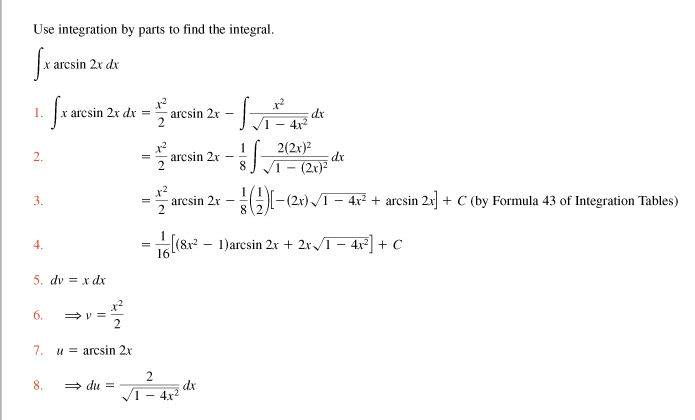# Integration by parts & inv. trig fxn

## Homework Statement

$$\int xarcsin2xdx$$

2. The attempt at a solutionCan someone explain to me what is happening at step 2? I understand how the integration by parts was done, but where does the (1/8) or (2x) come from?

## Answers and Replies

They multiplied the numerator and denominator by 8. Thus

$$\frac{x^2}{\sqrt{1-4x^2}}=\frac{8x^2}{8\sqrt{1-4x^2}}$$

and then they rewrote this slightly.

They multiplied the numerator and denominator by 8. Thus

$$\frac{x^2}{\sqrt{1-4x^2}}=\frac{8x^2}{8\sqrt{1-4x^2}}$$

and then they rewrote this slightly.

I still dont see how this gets (1/8), and even then, what is the purpose of doing this, and where does the (2x) come from?

Yoi get the 1/8 by bringing the denominator out of the integral.
And the numerator is rewritten as $8x^2=2(2x)^2$.

The purpose of doing that is to do a substitution t=2x.

Yoi get the 1/8 by bringing the denominator out of the integral.
And the numerator is rewritten as $8x^2=2(2x)^2$.

The purpose of doing that is to do a substitution t=2x.

Oh, ok. Thanks again!